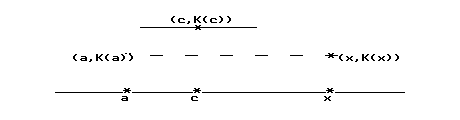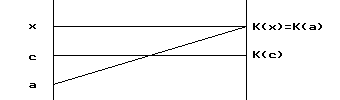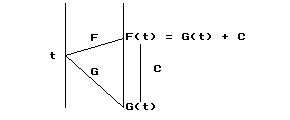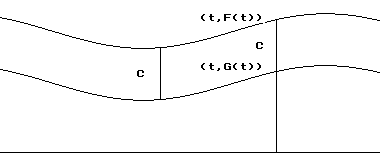﻿ Sensible Calculus IV.B
IV.B.1. Solving Differential Equations: Beginning Theory.
Jan., 2006: This page now requires Internet Explorer 6+ MathPlayer or Mozilla/Firefox/Netscape 7+.
Preface
: Think about a freight car and a caboose on a model electric train. Certainly as the train moves along, these two cars will be traveling at the same velocity. Now think about two model electric trains traveling on the same track so that at any given instant they are traveling at the same velocity. Will the distance between the two train engines always be the same?

Let's restate these contexts using the derivative and the question in terms of solutions to differential equations. Think of the position of the cars (or trains) as variables y and z that depend on time t. In the first context, where z is the freight car and y is the caboose, the context indicates that at any time, z(t) = y(t) + C where C is a constant. The derivative calculus justifies the fact that z'(t) = y'(t) at any time.

The second context with the two separate trains is different. It has as its hypothesis that z'(t) = y'(t). The question asks whether z(t) - y(t), which represents the distance between the two trains, will be a constant. You might agree it is a constant after thinking further about the train context. For the distance between the two trains to change, one train would have to go faster than the other for some time (however short). This would contradict the hypothesis that the two trains always travel at the same rate.

The example of Section IV.A described the general solution to the differential equation dy/dx = x 2 as the family of functions of the form y = x3 / 3 + C where C is a constant. This means that any two members of this solution family will differ only by a constant. Graphically then the family will have members that are parallel curves in the sense that any pair will have a constant vertical distance between them.

The next results clarify the consequences of two functions having the same derivative.. They also provide a scheme for describing general solutions to any differential equation of the form dy/dx = P(x), i.e., describing `int P(x) dx`. [N.B. The variable y does not control the derivative dy/dx in this last equation.]

THEOREM 4.1: Suppose K'(t) = 0 for all t in an interval I. Then K(t) = C for all t in I, i.e. K is a constant function on I.

Proof: Consider two distinct points in the interval, a and x, with a < x. The function K is differentiable on (a,x) and continuous on [a,x], i.e., K satisfies the hypotheses of the Mean Value Theorem for the interval [a,x]. Thus there is a point c between a and x where

K'(c) (x-a) = K(x) - K(a). (See Figure IV.B.i.)

But the  derivative assumption forces K'(c) = 0 = K(x) - K(a), so K(x) = K(a). This argument is true for any choice of x, so for all x in I, K(x) = K(a). Now we let C = K(a) and change the variable x to t to complete the argument that K is a constant function on I. EOPa. graph of Kb. transformation figure for K.
Figure IV.B.i

Interpretation: 1. In the interpretation of position and velocity, if the velocity is always 0, then the object stays in the same position.

2. In the tangent interpretation, if all the tangents to the graph of a function have a slope of 0, then the graph is in fact a horizontal line.

Comment: This theorem can be summarized by the statement, "Derivative 0 implies the function is a constant."

The next theorem states the result that  applies to the example differential equation dy/dx = x2 justifying the claim that the general solution is y = x3 / 3 + C.

THEOREM 4.2: If F'(t) = G'(t) for all t in an interval I, then F(t) = G(t) + C for all t in I.
Proof: The idea here is quite important. If we wish to compare two functions, it is sometimes helpful to consider their difference.
Let K(t) = F(t) - G(t). Then by the assumption,

K'(t) = F'(t) - G'(t) = 0 for all t in the interval.

Applying Theorem 4.1 to the function K shows there is a number C so that

C = K(t) = F(t) - G(t) for all t in the interval.

Now simple algebra shows that F(t) = G(t) + C for all t in I. EOP

Interpretation. 1. In the interpretation of position and velocity as suggested in the preface to this section, if the velocity of two moving objects is the same at every instant, then the objects have a constant distance between them as they move. [See Figure IV.B.i.]Figure IV.B.iFigure IV.B.ii
2. In the tangent interpretation, if for any given first coordinate the tangents to two curves always have the same slope, then the curves are parallel in the sense that there is a constant vertical distance between them. [See Figure IV.B.ii.]

Comment: This theorem can be summarized by the statement, "Functions with the same derivative differ by a constant."

CAVEAT: Both Theorems 4.1 and 4.2 should be distinguished from the following statements:

(i) If K(t) = C then K'(t) = 0, and
(ii) If F(t) = G(t) + C for all t in I then F'(t) = G'(t).

These two statements are immediate consequences of the definition of the derivative. Theorems 4.1 and 4.2 are the converses of these statements and we have relied on the Mean Value Theorem for their proof. The statements (i) and (ii) move from information about the functions to information about the derivatives. The theorems move from information about the derivatives of the functions over an interval to information about the functions themselves.

Applying Theorem 4.2 to the differential equation dy/dx = x 2 of section IV.A justifies our claim that the family of its solutions has the form y = x 3 /3 + C. The next application illustrates a similar situation.

Application: Find all functions F where F'(t) = -32t.

Solution: The function G(t) = -16t 2 has G'(t) = -32t. Now applying Theorem 4.2, any other function F with F'(t) = G'(t) has the form G(t) + C for some constant C. Thus any function F that satisfies F'(t) = -32t must have the form F(t) = -16t 2 + C for some constant C.

Part 2 of this section discusses the relation of Theorem 4.2 to indefinite integrals.

IV.B.2. The Calculus of Indefinite Integrals: Basic Facts.

Preface: The calculus of indefinite integrals (or antiderivatives) corresponds closely to the calculus of derivatives. If y is any member of the family of functions that is the indefinite integral of P then by the definition of this family, dy/dx = P(x). So, to check that you have found a member of this family, you need only differentiate your candidate function y and see that the derivative is P(x), the integrand.

[Keep in mind that the indefinite integral of P is a way to describe a family of functions characterized by solutions to the differential equation, dy/dx = P(x). This differential equation has the derivative depending only on one controlling variable. We do not use the indefinite integral to describe the general solution to an equation like dy/dx = x/y, because in this equation the derivative depends on both x and y.]

Developing a calculus for finding indefinite integrals uses this basic fact repeatedly. Unfortunately, the resulting calculus is not as easy or as successful in obtaining results symbolically when compared with the derivative calculus. What does this means in the interpretation of motion, where we thought we could just reproduce trips based on the velocity information? The difficulty in some situations is that even though we may be able to reproduce the trip, it is not always easy or even possible to find elementary formulae for the position of a moving object, even when the velocity is given by an elementary formula.

Fortunately it is easy to find indefinite integrals for polynomial functions. The task for rational functions (quotients of polynomials) is harder, though in theory there is a method for all rational functions. But for as simple a function as sin(x2), mathematicians have demonstrated there can be no elementary function F with F'(x)=sin(x2). Despite the difficulties that are present in describing indefinite integrals of elementary functions with elementary functions, there are some essential methods for finding them in common situations. This section begins the development of the calculus of indefinite integrals which will be continued further in later chapters.

The Constant of Integration (Application of Theorem 4.2 to the indefinite integral): If you have managed to find one member of the indefinite integral family for the integrand P, you have found a function F where F'(t)=P(t) on an interval. The theory in IV.B.1 is just what is needed to describe any other member of the family in terms of F. Here's how it works. If G is another member of the family for the same interval, then G'(t) = P(t) = F'(t) on that interval and Theorem 4.2 can be applied so that G(t)=F(t) + C on the interval. We summarize this result using the notation of the indefinite integrals:

If F'(t) = P(t) then  `int P(t) dt = F(t) + C` .

This result is sometimes treated as the definition of the indefinite integral. It leads to the following equations based only on the single function F.

`int F' (t) dt = F(t) + C`; `int DF(t) dt =  F(t) + C`  and  `int d/{dt}F(t) dt = F(t) + C`.

Convention: Recall that the letter "C" (or any other letter used to represent a constant in these formulae) is called the constant of integration. It is important to include this constant in a formula for the indefinite integral of P indicating that the indefinite integral is not just a single function, but a description of the family of all functions that satisfy the differential equation `{dy}/{dx} = P(x)`.

Example IV.B.2.1: Verify
(i)`int 3x^2 + 5 cos(x) + x sin(x) dx = x^3 + 6sin(x) - x cos(x) + C`
and  (ii)  `int  e^{5x} + ln(x) dx  = 1/5 e^{5x}  +  x ln(x)  -  x +  C` .

Check: These statements are verified [using the product rule and the chain rule!] by seeing that

` D_x (x^3 + 6sin(x) - x cos(x) ) = 3x^2 + 5cos(x) + x sin(x)`
and
`D_x ( 1/5 e^{5x} + x ln(x) - x + C)  =  e^{5x} + ln(x)`.

Since the indefinite integral consists of many functions described in a family characterized by describing any one member, it is helpful to develop a little better understanding of these families and some algebraic relations between them.

For example, let's consider the relation between  `int 5 x^2 dx` and `int x^2 dx`. It is not difficult to see that

`int 5 x^2 dx = 5/3 x^3 + C_1` while `int x^2 dx =1/3 x^3 + C_2`.
It is important to distinguish the constants used to represent the solutions here because it is certainly quite possible for these to be different numbers in solving a particular differential equation. Despite this difference in the solution's constants, we can say that any member of the family of solutions for  5 x2  would be 5 times a member of the family of solutions for  x2 . It is also true that 5 times any member of  the integral family for x2  would be a member of  the integral family of 5 x2. [Essentially this is because 5 times a constant is still a constant.] This relation between the functions identified by these two integrals is described by saying the integral of 5x2 is 5 times the integral of x2. This statement is written as an equality between integrals:
`int 5 x^2 dx = 5 int x^2 dx`.

We can consider in a similar fashion the relation between the integrals

`int 3x^2 dx = x^3 + C_1` , ` int 4x^3 dx = x^4 + C_2 ,` and `int 3 x^2 + 4x^3 dx = x^3 + x^4 + C_3`.

Here too the relation is quite simple because the sum of two constants is still a constant. The relation between these families is expressed by the equation

`int 3x^2 dx +  int 4x^3 dx = int 3x^2 + 4x^3 dx` .

We'll follow the notational convention of these examples for constant multiples and sums for the families of functions described by the indefinite integral.

A Calculus for Indefinite Integrals: One of the goals in solving problems is to develop procedures for finding solutions that minimize the need for lengthy arguments and investigations. This was a major achievement for the problem of finding the derivative. The procedure as we have seen in our previous work is called the calculus of derivatives or the differential calculus. The key organization of that calculus was two fold: (i) knowledge of the derivatives for a list of core functions and (ii) a collection of rules that allowed for the determination of a function's derivative based on the way it was assembled from the list of core functions.

We begin here to organize a similar calculus for indefinite integrals. Note that this calculus is not as comprehensive nor as successful as the derivative calculus. The integral calculus does not provide a procedure to determine a function's antiderivative for such relatively simple functions as `sin(x^2)`. The organization for the integral calculus is similar to that of the derivative calculus, with a list of results for core functions and a list of rules.

Here are some initial pieces of the calculus of indefinite integrals. These can be justified without much trouble using corresponding derivative statements.

 Type of Function Integral Solution Derivative Solution Power of x:  `x^p` `int x^p dx = 1/{p+1}x^{p+1} + C` [p not equal to -1] `d/{dx} x^p = p x^{p-1}` Trigonometric:  sin(x)  cos(x)  sec(x)tan(x) `sec^2 (x)` `int sin(x) dx = - cos(x) + C` `int cos(x) dx = sin(x) + C` `int sec(x) tan(x) dx = sec(x) + C` `int sec^2(x) dx = tan(x) + C` `d/{dx} sin(x) = cos(x)` `d/{dx} cos(x) = -sin(x)` `d/{dx} tan(x) = sec^2(x)` `d/{dx}sec(x) = sec(x) tan(x)` Exponential: `e^x` `int e^x dx = e^x + C` `d/{dx} e^x = e^x`

 IV.B.2 (Constant Multiples) `int alpha P(x) dx = alpha int P(x) dx` `D_x[ alpha F(x)] = alpha D_x [F(x)]` IV.B.3 (Sums) `int P(x) + Q(x) dx = int P(x) dx  + int Q(x) dx` `D_x[ F(x) + G(x) ] = D_x [F(x)] + D_x [G(x) `

Note:
In IV.B.1 the equation `int x^p dx = 1/{p+1} x^{p+1} + C ` applies to all powers p except p = -1. When p = -1 the formula does not make sense since then the denominator p+1 is equal 0.

Here's the proof of IV.B.3 as an example of how these proofs are done:

Suppose `int P(x)dx = F(x) + C_1` and  `int Q(x)dx = G(x) + C_2`.
Then `DF(x) = P(x)` and `DG(x) = Q(x)`.
Thus `D [F(x)+G(x)] = P(x) + Q(x)`, so that

`int P(x)dx + Q(x)dx  = F(x) + G(x) + C_3`.
Now any member of the indefinite integral family of P when added to a member of the indefinite integral family for Q will have the form F(x) + G(x) + C4 (a constant).
This means that  `int [P(x)+Q(x)]dx = int P(x)dx +  int Q(x)dx`. EOP

COMMENT: These basic facts for the calculus of indefinite integrals are sometimes described as follows:

IV.B.1 is called "the power rule" for integrals or "the up-one rule" for integrals.

IV.B.2 is called "the constant (or scalar) multiple rule" or "the homogeneity property" for integrals.

IV.B.3 is called "the sum rule" for integrals.

IV.B.2 and IV.B.3 are referred to together as "the linearity properties" of the indefinite integral.

EXAMPLE: Find `int 5x ^ 3 + 3x^ 2 - 5 dx` .

Solution: Begin by applying IV.B.3 to distribute the integral across the sum so that

`int 5x^ 3 + 3x^ 2 - 5 dx = int 5x^ 3 dx + int 3x^ 2 dx - int 5 dx` .

Now apply IV.B.2 to each of the summands to bring the constants in front of each integral so that

`int 5x^ 3 + 3x^ 2 - 5 dx = 5 int x^ 3 dx +  3 int x^ 2 dx - 5 int 1  dx` .

Finally we use IV.B.1 to find the integrals of the powers of x and 1 (= x 0):

`int 5x^ 3 + 3x^ 2 - 5 dx = 5/4 x^ 4 dx +  3/3 x^ 3 dx - 5x + C` .

This solution can be verified by differentiating `y = 5/4 x^ 4 + x^ 3 - 5x`. This is left for the reader.

COMMENT: Finding the indefinite integral for a polynomial function is quite elementary. Unfortunately, as mentioned in the preface, not all functions have such elementary indefinite integrals. You can investigate more of the calculus of indefinite integrals in some of the exercises for this section. A more detailed treatment of integration appears in chapter VII. In the next sections of this chapter we will consider more physical, visual, and numerical aspects of differential equations. These other viewpoints should help increase your understanding of what it means to solve a differential equation.
Visualizing the graphs of integrals: Click here to see a sketch that allows you to see the relation between the graph of  f (x) = A + Bx +  Cx2 +Dx  and its integral P(x) =  K +Ax + Bx2/2+ Cx3/3 +D x4 /4

PROBLEMS IV.B.

For problems 1 to 8 find the general solution for the given differential equation.

1. y' = 3x 2 - 2x + 5.

2. Df(x) = x 2 + 3x + 5.

3. dy/dt = cos(t) + sin(t).

4. f'(x) = 3x 3 + sec 2(x) - 1/x 2 .

5. dz/dt = 12t 2 -sin(t) + 2 - 5/(1+ t) 2 .

6. g'(u) = 5 sin(u) - 10 cos(u).

7. r'(q) = e3q.

8. w' = 12x(5+ x 2)10.

9. Find the general solution for the differential equation y' = 3x 2 + 5x - 1.

For each of the following conditions give a solution to this differential equation:

(a) y(0) = 7 (b) y(0) = -4 (c) y(1) = 7 (d) y(1) = -4

10. Find each of the following indefinite integrals.

 (a) `int 3t + 4 - 5/{t^ 7 }dt` (b)`int x^3 + 4x^5 dx ` (c) `int 4 y^{ -3} + sin(y) dy` (d) `int t ^{1/3} - sec(t)tan(t) dt` (e) `int - 1/2 x + x ^{1/2 }dx` (f) `int 3 sec^2 (q) + 2/{sec(q)} dq`
11. Prove IV.B.1.

12. Prove IV.B.2.

13. Polynomials: Describe a procedure for finding the indefinite integral of a polynomial function that could be used by a student who has just learned the algebra needed to describe a polynomial function.

14. Prove: `int P(x) Q'(x) dx +  int Q(x) P'(x) dx = P(x) Q(x) + C`. [Hint:Use linearity and the product rule for derivatives.]

15. Use problem 14 to find (i) `int xcos(x)dx` and (ii)  `int  xe^x dx`. [Hint: Use P(x) = x and Q(x)=sin(x) or Q(x)=ex.]

16. Use problems 14 and 15 to find (i) `int x^ 2 sin(x)dx` and (ii) `int  x^2e^x dx`. [Hint: Use `P(x) = x^ 2`.]

17. Prove `int P'(Q(x)) Q'(x) dx = P(Q(x)) + C`. [Hint: Use the Chain Rule.]

18. Use problem 17 to find  `int 3 cos^2(x) sin(x) dx`. [Hint: Use P(t) = t 3 and Q(x) = cos(x).]

19. Use problem 17 to find ` int 3x^ 2 (x^ 3+5)^10 dx`. [Hint: Use P(t) = t 10 and Q(x) = x 3 +5.]

20. Suppose an object is moving on a straight line with its velocity determined by the function v(t) = t2 + t feet per second at time t seconds. If the object is located with its position at 10 feet to the right of the 0 foot marker at 2 seconds, where will the object be at 3 seconds? Where was the object at time 0?

21. Verify by differentiation that  `int 2 sin(x) cos(x) dx = sin^2(x) + C` and

`int  2 sin(x) cos(x) dx = -cos^2(x) + C`. Thus sin2(x) + C = -cos2(x) + C. Using algebra and trigonometry this gives 1 = sin2(x) + cos2(x) = C - C = 0. Obviously this last statement is not true. Find the error made in reaching this conclusion.